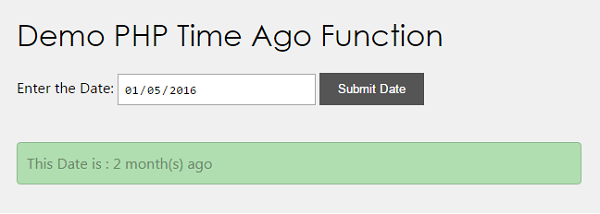# PHP Time Ago Function

This PHP tutorial is for converting given date into a time ago string like 2 hours age, 3 years ago. In a previous tutorial, we have seen date format conversion by using PHP inbuilt functions.

In this example, we are having a custom function to change a date format into time ago string.

View Demo

## HTML Date Input

This HTML will show a form with a date field to get a date from the user.``````<form name="frmTimeAgo" method="post">
Enter Date:
<input type="date" name="date-field" value="<?php if(!empty(\$_POST["date-field"])) { echo \$_POST["date-field"]; } ?>"/>
<input type="submit" name="submit-date" value="Submit Date" >
</form>
<?php
if(!empty(\$strTimeAgo)) {
echo "Result: " . \$strTimeAgo;
}
?>
``````

## PHP Custom Time Ago Function

This PHP code reads the value of the date field on the form post. This value will be passed as an argument to the custom timeago() function.

In timeago() function the given date is converted into timestamp using PHP built-in strtotime(). And, this timestamp is subtracted from the current timestamp to calculate elapsed time.

The time elapsed from the given date till now is used to calculate the time ago string. The code is,

``````<?php
\$strTimeAgo = "";
if(!empty(\$_POST["date-field"])) {
\$strTimeAgo = timeago(\$_POST["date-field"]);
}
function timeago(\$date) {
\$timestamp = strtotime(\$date);

\$strTime = array("second", "minute", "hour", "day", "month", "year");
\$length = array("60","60","24","30","12","10");

\$currentTime = time();
if(\$currentTime >= \$timestamp) {
\$diff     = time()- \$timestamp;
for(\$i = 0; \$diff >= \$length[\$i] && \$i < count(\$length)-1; \$i++) {
\$diff = \$diff / \$length[\$i];
}

\$diff = round(\$diff);
return \$diff . " " . \$strTime[\$i] . "(s) ago ";
}
}

?>
``````